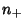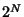## Fisher Sign Test

A robust nonparametric test which is an alternative to the Paired t-Test. This test makes the basic assumption that there is information only in the signs of the differences between paired observations, not in their sizes. Take the paired observations, calculate the differences, and count the number ofsands, whereis the sample size. Calculate the Binomial CoefficientThengives the probability of getting exactly this manys ands if Positive and Negative values are equally likely. Finally, to obtain the P-Value for the test, sum all the Coefficients that areand divide by.